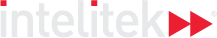### Course Overview

Trigonometry 1 provides an introduction to right angled triangles, and the relationships between the sides and angles. The Pythagorean Theorem, which the students have studied in earlier math courses, is shown in context, and the basic trigonometric functions are defined and explained. The final lessons discuss the trigonometric functions of special angles as well as how to graph all of these functions.

HOURS OF INSTRUCTION: 15

### Course Outline

• Trigonometric Functions
• Pythagorean Theorem
• Pythagorean Theorem and Trigonometric Identities
• Values of Trigonometric Functions for Special Angles
• Graphs of Trigonometric Functions